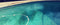This guide explains how to remove the chlorine smell from your pool. When you're around the swimming pool, you shouldn't really smell much of anything. A chlorine smell is not a good thing as people may think. It is actually the presence of chloramines which may indicate a larger problem like chlorine lock.

1. If you have a strong smell of chlorine coming from your pool it is actually not the smell of chlorine, but rather the smell of chloramines. Chloramines are the result of a chemical compound of chlorine and ammonia. It is toxic in nature and is poisonous to animals and fish. A chloramine issue has to be dealt with a certain way because it can not evaporate or dissipate the way chlorine does. Make certain that no one is swimming in the pool until the chloramines have been removed.

2. The presence of chloramines is usually an indication that your pool water is not in the correct balance. You more then likely have a higher than normal chloramine levels. This is what gives off that chlorine smell as well as causes skin and eye irritation.

3. There are a couple of ways to get rid of the chlorine smell. The first way is to use a chlorine based shock to the pool. You will need to reach what is called "break point chlorination" to get rid of the smell. Breakpoint chlorination is the point where the chemical bond that ties nitrogen, chlorine and ammonia together by using large amounts of chlorine. This will cause the chlorine residual to drop rapidly. Reaching a true chlorine breakpoint is vital to breaking a chlorine lock. If breakpoint is not reached using this method, the problem will only get worse. To accomplish a breakpoint, 7.6 free chlorine molecules are used to break apart an individual combined chlorine molecule. The amount of product you will need will vary depending on the type of shock you are using. Aside from knowing your total chlorine, free chlorine, number of gallons in the pool, and the weight of a gallon of water (8.34 lbs.), you will also need to know the type of pool shock you are using. Before attempting this method, the pH needs to be between 7.2-7.4. This equation will be a two part process. The first step will be determining the ppm of chlorine needed to reach breakpoint: (TC-FC) x 10. Using the same amount above, the equation would look like this: (5-3) x 10 = x ppm or 2 x 10 = 20 ppm. I will need 20 ppm of chlorine to reach breakpoint in this 15,000 gallon pool. The next step is to determine how much product I will need. I'm using calcium hypochlorite in this example and need 2 oz. per 10,000 for a 1 ppm increase. I'll need to multiply 2 oz. x (gallons in pool/10,000) x (ppm needed/ppm dosage) which simply put is: 2 oz. x (15,000/10,000) x (20 ppm/1 ppm) or 2 oz x 1.5 x 20= 60 oz. To convert ounces to pounds, I divide by 16: 60 oz./16= 3.75 lbs. So to reach breakpoint in my 15,000 gallon pool I will need 3.75 lbs. of calcium hypochlorite.

4. The other way to remove chloramines from the pool is to use a non chlorine based shock. The non-chlorine shock will need to be added in similar fashion as step 3. To determine the amount of non-chlorine shock you will need, you will need to do some math. You will need to subtract the free chlorine from the total chlorine and multiply that by the number of gallons in the pool divided by 10,000 then multiply by 2. To make it easy it looks like this: (TC-FC) x (#of gallons/10,000) x 2 = amount of non-chlorine shock. If we use a total chlorine reading of 5 ppm and a free chlorine of 3 ppm on a 15,000 gallon pool, the equation would look like this: (5-3) x (15,000/10,000) x 2 = x. Therefore: 2 x 1.5 x 2 = 6. I would need to add 6 lbs. of a non-chlorine shock to the pool.

5. Once the chlorine smell is gone, you can use a product like Pool First Aid or Pool Perfect as part of your maintenance to help break down the organic waste that contributes to the presence of chloramines.

If you have any other questions about pool and spa products please do let us know - we are here to help!